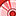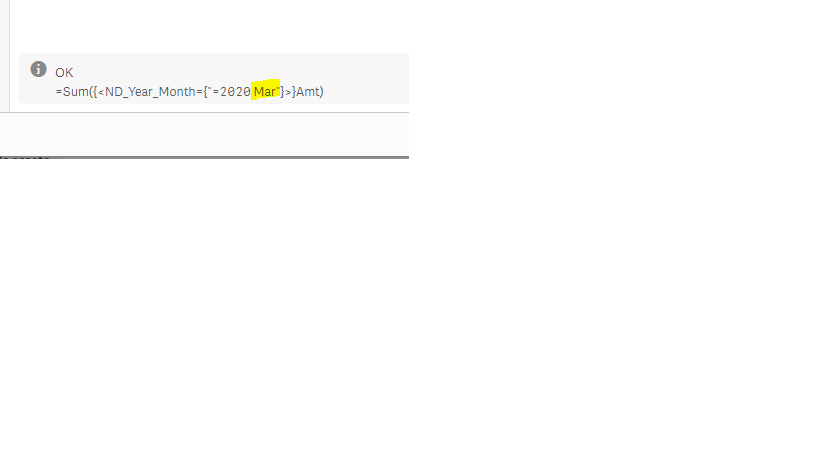# New to Qlik Sense

If you’re new to Qlik Sense, start with this Discussion Board and get up-to-speed quickly.

Announcements
QlikWorld 2023, a live, in-person thrill ride. Save \$300 before February 6: REGISTER NOW!
cancel
Showing results for
Did you mean:Contributor III

## Set Analysis - Combine two values for one set modifier

Hello everyone

I'm trying to write a set analysis where I only get the sum from those ND_Presence fields where the ND_Year_Month is equal to the year of ND_Test, space, month of ND_Test.I've tried multiple syntaxes, but can't seem to make it work in a set analysis

sum( {< ND_Year_Month = {"=\$(=year(ND_Test))"} &' '& {"=\$(=month(ND_Test))"}}>} ND_Presence)

Labels (3)

• ### set modifier

2 Solutions

Accepted SolutionsSpecialist III

Try sum({<ND_Year_Month={"=\$(=year(ND_Test)&' '&month(ND_Test))"}>}ND_Presence)

Also in the expression editor, verify the results of the equation at the bottom.  In my test, the month is in camel case.Contributor III
Author

It works if I delete the after the opening double quotes! Thank you so much!

sum({<ND_Year_Month={"\$(=year(today())&' '&month(today()))"}>}ND_Presence)

2 RepliesSpecialist III

Try sum({<ND_Year_Month={"=\$(=year(ND_Test)&' '&month(ND_Test))"}>}ND_Presence)

Also in the expression editor, verify the results of the equation at the bottom.  In my test, the month is in camel case.Contributor III
Author

It works if I delete the after the opening double quotes! Thank you so much!

sum({<ND_Year_Month={"\$(=year(today())&' '&month(today()))"}>}ND_Presence)Tags
Community Browser